# Reconstruct Ground Truth Trajectory from Sampled Data Using Filtering, Smoothing, and Interpolation

This example shows how to use an interactive motion-model based filter along with a smoother to reconstruct the ground truth trajectory based on interpolation techniques.

### Using Tracking Filters

Many trajectories can be segmented into a series of discrete maneuvers across time, where each maneuver is governed by a small set of parameters that control how the pose of the object changes over time. These maneuvers often consist of very simple models, for example, a constant velocity, constant turn, or constant acceleration model. These simple models can be used with a tracking filter that is tolerant to small perturbations of the parameters.

While these perturbations should be small, they do not necessarily need to be random. For example, a constant velocity filter can often track an object with a small constant acceleration at the expense of a small bias in the tracking result. Filter performance can drop severely when these perturbations are significant.

In the Tracking Maneuvering Targets example, you learn how to use an interacting multiple-model (IMM) filter to track a target whose trajectory can be divided into three segments: a single constant velocity, a constant turn, and a constant acceleration segment. The IMM filter is a “hybrid” filter that runs a set of filters in parallel and returns a result that weights the estimates of each filter by its performance. You can see the result of the example reproduced below:

```n = 1000; [trueState, time, fig1] = helperGenerateTruthLoop(n); dt = diff(time(1:2)); numSteps = numel(time); rng(2021); % for repeatable results % Select position from 9-dimenstional state [x;vx;ax;y;vy;ay;z;vz;az] positionSelector = [1 0 0 0 0 0 0 0 0; 0 0 0 1 0 0 0 0 0; 0 0 0 0 0 0 1 0 0]; truePos = positionSelector*trueState; sigma = 10; % Measurement noise standard deviation measNoise = sigma* randn(size(truePos)); measPos = truePos + measNoise; initialState = positionSelector'*measPos(:,1); initialCovariance = diag([1 1e4 1e4 1 1e4 1e4 1 1e4 1e4]); % Velocity and acceleration are not measured detection = objectDetection(0,[0; 0; 0],'MeasurementNoise',sigma^2 * eye(3)); f1 = initcaekf(detection); % Constant acceleration EKF f1.ProcessNoise = 0.3*eye(3); f2 = initctekf(detection); % Constant turn EKF f2.ProcessNoise = diag([1 1 100 1]); f3 = initcvekf(detection); % Constant velocity EKF imm = trackingIMM({f1; f2; f3},'TransitionProbabilities',0.99, ... 'ModelConversionFcn',@switchimm); initialize(imm, initialState, initialCovariance); estState = zeros(9,numSteps); for i = 2:size(measPos,2) predict(imm,dt); estState(:,i) = correct(imm,measPos(:,i)); end figure(fig1); ax = gca(fig1); line(ax,estState(1,:),estState(4,:),estState(7,:),'Color','k','LineStyle',':','DisplayName','Correction estimates'); axis(ax,'image');```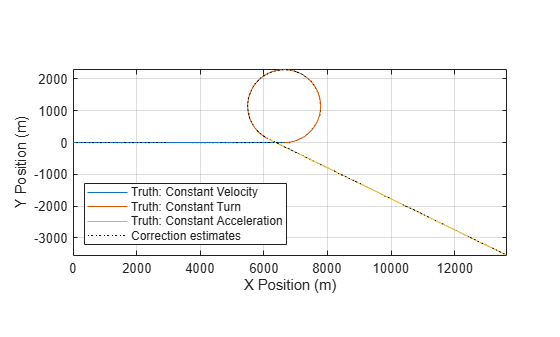Zoom into the transition regions between each model:

```axis(ax,[6000 7000 -200 200]) legend(ax,'Location','bestoutside')```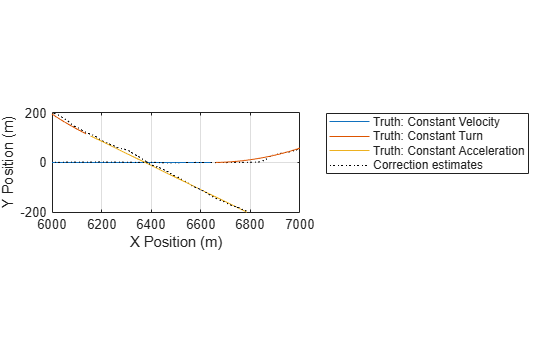The IMM filter faces challenges in the time intervals after the motion model changes from one to another. In each transition region the centripetal acceleration is not accounted for in the constant velocity and constant acceleration models. Therefore, the estimation bias persists for a small duration until the IMM filter has enough data to determine the correct motion model. The bias vanishes thereafter until the next transition region.

### Smooth Trajectory Estimates

Smoothing is an effective technique to retroactively improve the trajectory estimates. Smoothing is performed by applying both a forwards and backwards filter. The smoother can then choose the "best" of the forwards and backwards predicted regions that mitigate prediction errors in the transition region between maneuvers. You use the same data and filter setup as above, but with the smoothing capability enabled.

```imm = trackingIMM({f1; f2; f3},'TransitionProbabilities',0.99, ... 'ModelConversionFcn',@switchimm, ... 'EnableSmoothing',true, ... 'MaxNumSmoothingSteps',size(measPos,2)-1); initialize(imm, initialState, initialCovariance); estState = zeros(9,numSteps); for i = 2:size(measPos,2) predict(imm,dt); estState(:,i) = correct(imm,measPos(:,i)); end smoothState = smooth(imm); line(ax,smoothState(1,:),smoothState(4,:),smoothState(7,:),'Color','g','LineStyle','-','DisplayName','Smooth estimates') axis(ax,'image');```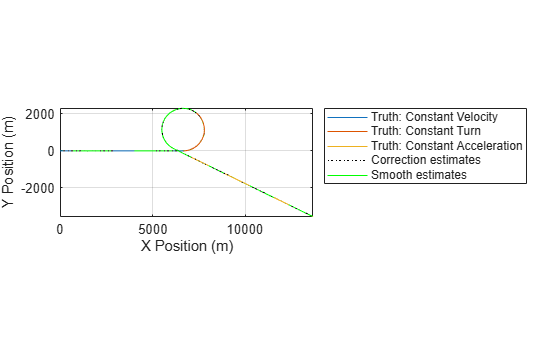Zoom in the same region as above and you can see that the smoothed estimates are considerably more successful at recovering the ground truth trajectory.

`axis(ax,[6000 7000 -200 200])`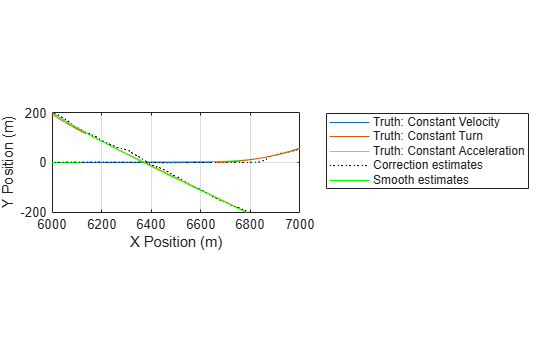From the results, you can see that smoothing is an effective tool to recover trajectory data when transitioning from one motion model to another with an abrupt change. This forward-backward smoothing often reasonably well in estimating a ground truth trajectory.

### Using Low-Order Polynomials for Densely Sampled Data

When comparing tracking algorithms, it can be advantageous to present the tracking results into a format so that pose information can be queried for an arbitrary time instant. When data is sampled at a sufficiently high rate, you can use a set of low-order piecewise polynomials to approximate the data. These polynomials can be differentiated to provide velocity and acceleration information. Additionally, you can use a set of algorithms to infer the orientation of an object based on certain assumptions. For example, assume an object always faces the direction of travel and banks to counteract centripetal forces. The following code shows how to use the smoothed positions and velocities to infer the total acceleration of the object by using a cubic spline.

```smoothPos = smoothState([1 4 7],:); smoothVel = smoothState([2 5 8],:); % Smoothed state vector does not include acceleration % Create piecewise polynomial from velocity information velPP = pchip(time(2:end),smoothVel); % Construct a new piecewise polynomial with the derivative [breaks,coefs,~,k,dim]=unmkpp(velPP); coefs = coefs(:,1:k-1).*(k-1:-1:1); accPP=mkpp(breaks,coefs,dim); % Evaluate the piecewise polynomial to get the acceleration smoothAcc = ppval(accPP,time(2:end)); % Display the velocity and resulting acceleration fig = figure; ax1 = subplot(2,1,1,'Parent',fig); plot(ax1,time(2:end),smoothVel(1,:),time(2:end),smoothVel(2,:),time(2:end),smoothVel(3,:)); xlabel('time [s]'); ylabel('velocity [m/s]') title('Velocity Profile') legend('v_x','v_y','v_z') ax2 = subplot(2,1,2,'Parent',fig); plot(ax2,time(2:end),smoothAcc(1,:),time(2:end),smoothAcc(2,:),time(2:end),smoothAcc(3,:)); xlabel('time [s]') ylabel('acceleration [m/s^2]') title('Acceleration Profile') legend('a_x','a_y','a_z')```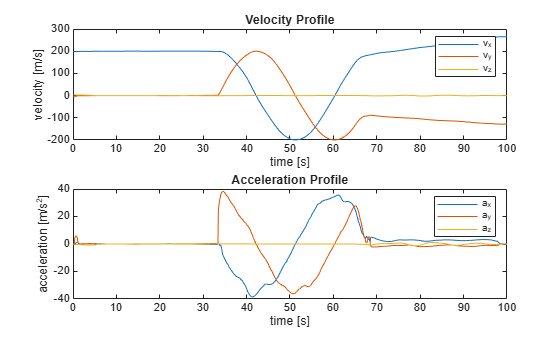The computed acceleration is not purely sinusoidal while in the constant turn maneuver, which is due to small variations in the smoothed output. Nevertheless, the result provides information to identify the distinct regions of each maneuver.

Though the cubic polynomials are numerically efficient to interpolate the position, they rely on having dense sampling to faithfully represent circular arcs. If greater accuracy is required, you need to use data sampled at a higher rate, which requires an increase of memory resources and a larger lookup table.

### Using High-Order Polynomials

In the previous section you used cubic polynomials to compute the acceleration at each point. For trajectories that consist mainly of circular and straight motion, using an interpolant that favors constant curvature works considerably better than low-order polynomials. The `waypointTrajectory` object can model trajectories with constant turn (constant curvature). It can also faithfully represent trajectories with gradual changes in both curvature and acceleration.

A simple technique to reduce the memory footprint of the interpolant and reduce the magnitude of transients is to use changepoint estimation to locate significant changes in curvature of the trajectory. After that, use those points to compute the interpolant.

```% Obtain curvature of trajectory projected into x-y plane planarCurvature = cross(smoothVel,smoothAcc) ./ vecnorm(smoothVel,2).^3; horizontalCurvature = planarCurvature(3,:); % Obtain the estimates of the changepoints threshold = var(horizontalCurvature); [tf,m] = ischange(horizontalCurvature,'Threshold',threshold); fig=figure; hAx=gca(fig); line(hAx,time(2:end),horizontalCurvature,'DisplayName','Computed'); [xx,yy]=stairs(time(2:end),m); line(hAx,xx,yy,'DisplayName','Estimated','Color','k') legend(hAx) xlabel('time [s]'); ylabel('curvature [rad/m]') title('Horizontal curvature')```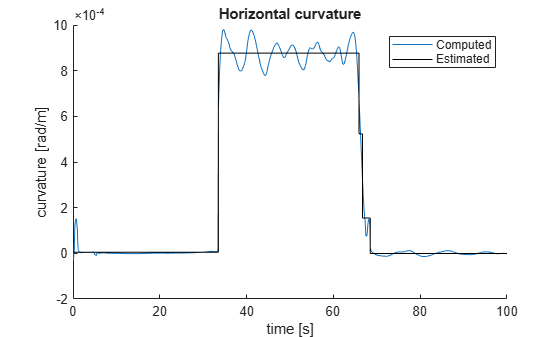You can then take these changepoints as starting points for constructing the waypoints of the trajectory:

```idx = 1+[0 find(tf) numel(tf)-1]; waypoints = smoothPos(:,idx)'; wayvels = smoothVel(:,idx)'; timeOfArrival = time(idx); traj = waypointTrajectory(waypoints,timeOfArrival,'Velocities',wayvels); [wayPos, ~, wayVel] = lookupPose(traj,time(1:end-1)); clf plot(trueState(1,:),trueState(4,:),'-'); hold on; plot(wayPos(:,1),wayPos(:,2),'-') plot(waypoints(:,1),waypoints(:,2),'o '); grid on; xlabel('X Position [m]'); ylabel('Y Position [m]'); axis equal; legend('Truth','Waypoint Estimate', 'Waypoints', 'Location','southwest')```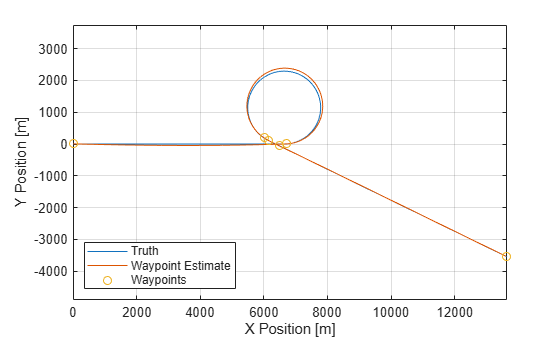From the results, you recovered a circular trajectory by using just a few waypoints and their corresponding velocities. However, the radius is sensitive to the tangent angles of the velocities estimated within the transition region - which can be a difficult region for a tracking filter to predict.

If you plot the magnitude of the position error of the reconstructed trajectory against the smoothed estimates, you can see localized regions with larger errors:

```figure; plot(time(2:end),vecnorm(smoothPos-wayPos',2)) xlabel('time [s]') ylabel('distance [m]') title('Magnitude of Position Error');```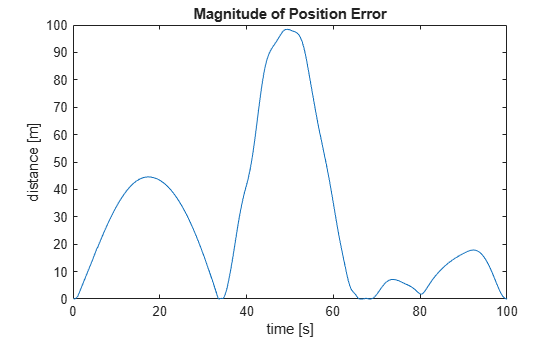Note the close agreement in regions where the waypoints are close to their smoothed counterparts and the larger deviations in the regions further away from the waypoints. A simple way to reduce the error is to repeatedly add additional waypoints at the positions of maximum error until a desired objective is achieved. In this example, if you set an objective of 10 meters for the maximum error, you only need a few more waypoints.

```objective = 10; % use 10 m for objective maximal position error maxError = Inf; % pre-assign error to a maximal value while maxError > objective [~,maxi] = max(vecnorm(smoothPos-wayPos',2)); idx = unique(sort([maxi idx])); waypoints = smoothPos(:,idx)'; wayvels = smoothVel(:,idx)'; timeOfArrival = time(idx); traj = waypointTrajectory(waypoints,timeOfArrival,'Velocities',wayvels); [wayPos, ~, wayVel, wayAcc] = lookupPose(traj,time(1:end-1)); maxError = max(vecnorm(smoothPos-wayPos',2)); end figure; plot(trueState(1,:),trueState(4,:),'-'); hold on; plot(wayPos(:,1),wayPos(:,2),'-') plot(waypoints(:,1),waypoints(:,2),'o '); grid on; xlabel('X Position [m]'); ylabel('Y Position [m]'); axis equal; legend('Truth','Waypoint Estimate', 'Waypoints', 'Location','southwest')```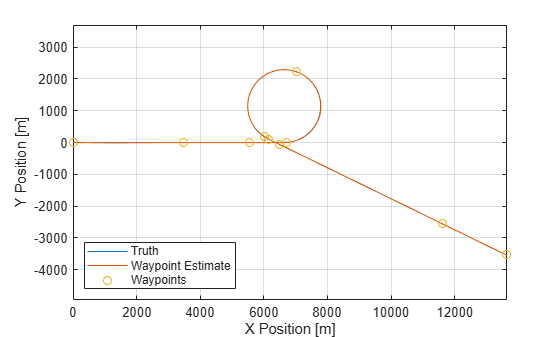You can then observe the reduced position error.

```figure; plot(time(2:end),vecnorm(smoothPos-wayPos',2)) xlabel('time [s]') ylabel('distance [m]') title('Magnitude of Position Error');```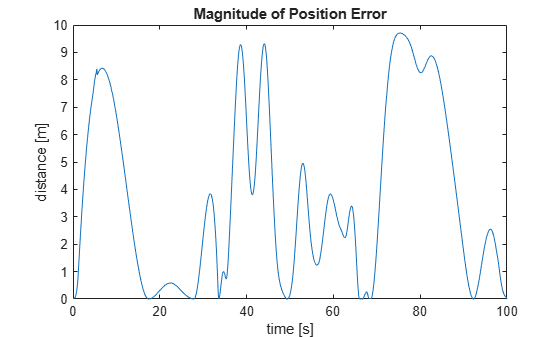Now that you have a reasonable position profile, you can inspect the resulting velocity and acceleration profiles from the trajectory. Note the overall improvement in the recovery of the sinusoidal acceleration profile.

```fig = figure; ax1 = subplot(2,1,1,'Parent',fig); plot(ax1,time(1:end-1),wayVel); title('Velocity Profile') xlabel('time'); ylabel('velocity [m/s]') legend('v_x','v_y','v_z') ax2 = subplot(2,1,2,'Parent',fig); plot(ax2,time(1:end-1),wayAcc); title('Acceleration Profile') xlabel('time [s]'); ylabel('acceleration [m/s^2]') legend('a_x','a_y','a_z')```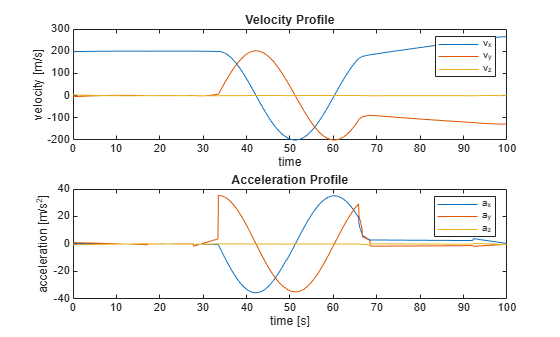### Summary

In this example you learned how to reconstruct a ground truth trajectory using an interacting multiple-model smoother and low order polynomials. You also used a simple scheme that used a high-order interpolant (contained in the `waypointTrajectory` object) along with critical sampling to recover higher-order derivatives that would otherwise be masked by noise.